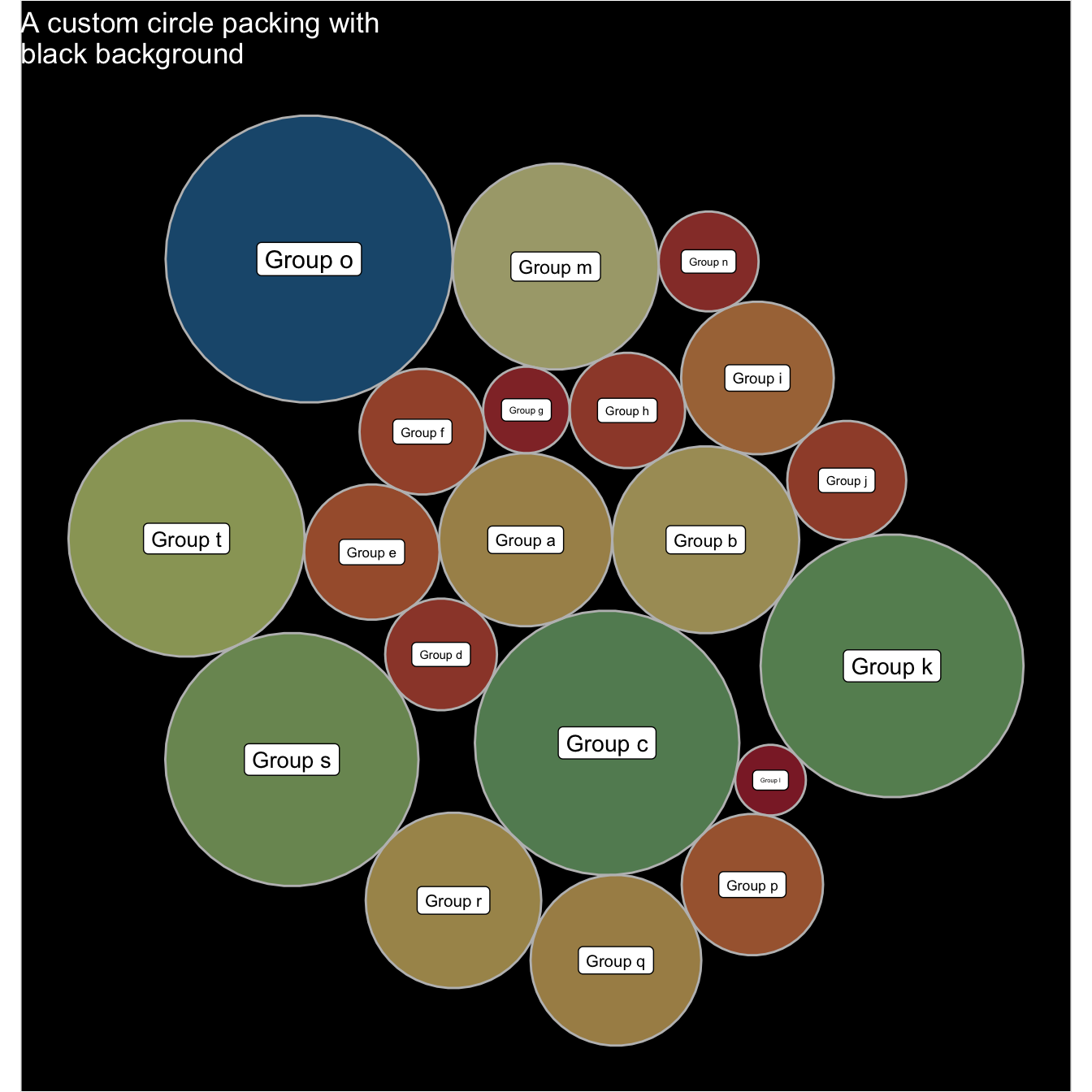# Circle packing customization with R

This page is dedicated to one level circle packing customization with R. It notably shows how to use different color palettes and provides reproducible code snippets.

# Using the `viridis` color scale

This chart follows the previous most basic circle packing post.

It shows how to use the awesome `viridis` package to build color scales, a very good alternative to the usual `colorBrewer`.

Note that `magma` is used here, but you could use the same code with `inferno` or viridis` instead.``````# libraries
library(packcircles)
library(ggplot2)
library(viridis)

# Create data
data <- data.frame(group=paste("Group", letters[1:20]), value=sample(seq(1,100),20))

# Generate the layout. sizetype can be area or radius, following your preference on what to be proportional to value.
packing <- circleProgressiveLayout(data\$value, sizetype='area')
data <- cbind(data, packing)
dat.gg <- circleLayoutVertices(packing, npoints=50)

# Basic color customization
ggplot() +
geom_polygon(data = dat.gg, aes(x, y, group = id, fill=as.factor(id)), colour = "black", alpha = 0.6) +
scale_fill_manual(values = magma(nrow(data))) +
geom_text(data = data, aes(x, y, size=value, label = group)) +
scale_size_continuous(range = c(1,4)) +
theme_void() +
theme(legend.position="none") +
coord_equal()``````

# Map color to bubble value

It is a common task to make the bubble color being lighter or darker according to its value.

This is possible by passing the focus variable to the dataframe that is read by `ggplot2`, and specifying it in tha `aes()`.``````# First I need to add the 'value' of each group to dat.gg.
# Here I repeat each value 51 times since I create my polygons with 50 lines
dat.gg\$value <- rep(data\$value, each=51)

# Plot
ggplot() +

# Make the bubbles
geom_polygon(data = dat.gg, aes(x, y, group = id, fill=value), colour = "black", alpha = 0.6) +
scale_fill_distiller(palette = "BuPu", direction = 1 ) +

# Add text in the center of each bubble + control its size
geom_text(data = data, aes(x, y, size=value, label = group)) +
scale_size_continuous(range = c(1,4)) +

# General theme:
theme_void()  +
theme(legend.position="none") +
coord_equal()``````

# Background customization

Change the background thanks to the `theme()` function and its `plot.background()` argument.``````ggplot() +

# Make the bubbles
geom_polygon(data = dat.gg, aes(x, y, group = id, fill=value), colour = "grey", alpha = 0.6, size=.5) +
scale_fill_distiller(palette = "Spectral", direction = 1 ) +

# Add text in the center of each bubble + control its size
geom_label(data = data, aes(x, y, size=value, label = group)) +
scale_size_continuous(range = c(1,4)) +

# General theme:
theme_void()  +
theme(
legend.position="none",
plot.background = element_rect(fill="black"),
plot.title = element_text(color="white")
) +
coord_equal() +
ggtitle("A custom circle packing with\nblack background")``````

Related chart types

## Contact

This document is a work by Yan Holtz. Any feedback is highly encouraged. You can fill an issue on Github, drop me a message on Twitter, or send an email pasting yan.holtz.data with gmail.com.